### Particle Swarm Optimization (PSO)## Introduction

xlOptimizer implements Particle Swarm Optimization (PSO), a a well-known swarm intelligence algorithm suitable for global optimization with no need for direct evaluation of gradients. The method, introduced by Kennedy and Eberhart , mimics the social behavior of flocks of birds and swarms of insects.

## How does it work?

PSO has many similarities with other algorithms such as Genetic Algorithms (GA). At first, the population (or "swarm") is initialized with random solutions. However, unlike GA, PSO has no selection or evolution operators such as crossover and mutation; in PSO the members of the swarm (or "particles") "fly" through the problem space following simple mechanics. Thus, PSO searches for optima by updating each particle according to notions such as position, velocity, inertia etc. This movement of particles is not entirely random; each particle is attracted towards both its own personal best position and the swarm's best position.

## Mathematical formulation

We assume that the population consists of p individuals. Within the Design Space (DS) each individual is characterized by its position and velocity, determined at time instant k by the corresponding vectors xk and vk. Initially, the particles are distributed randomly in the box-constrained search space, as follows: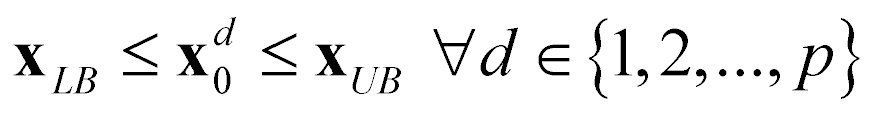where, xLB and xUB are vectors defining the lower and upper values of the variables, respectively. The position vector of individual d at the next time instant k+1 is given as: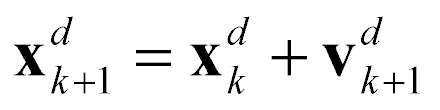where the time step Δt between the distinct time instants is assumed to be equal to unity. The velocity vector vdk+1 is given as: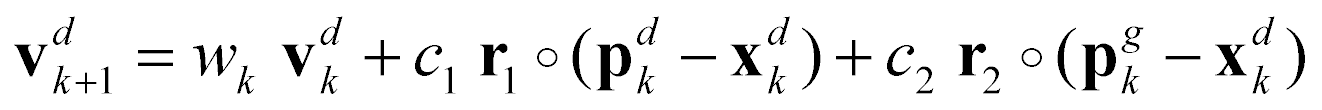where, wk is the inertia factor at time instant k; c1, c2 are the cognitive and social parameters of the algorithm, respectively; pdis the best ever position vector of individual d at time instant kpgk is the best ever position vector amongst all individuals at time instant k; r1, r2 are vectors of random variables with uniform distribution in the interval [0,1] and the (o) operator indicates element-by-element multiplication. The simple PSO algorithm imposes no limitations with regard to the maximum velocity of the particles.

Usual parameter values for small to medium-sized problems are the following: (a) population size p=20-30, (b) cognitive parameter c1=2, (c) social parameter c2=2, (d) constant inertia factor w=0.8 .

## Options in xlOptimizer

The following options are available in xlOptimizer:

#### Step 1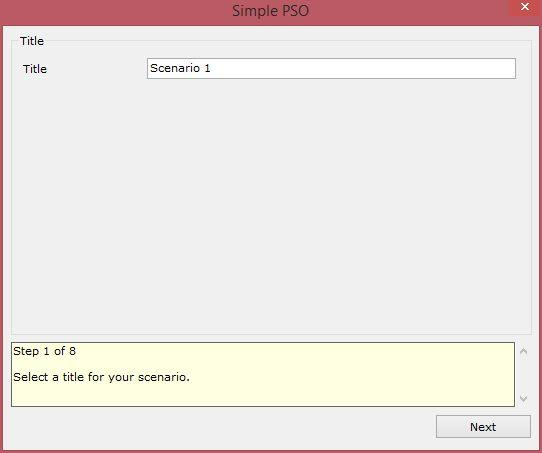Enter a short title for the scenario. The log files will be saved to a subfolder with this name.

#### Step 2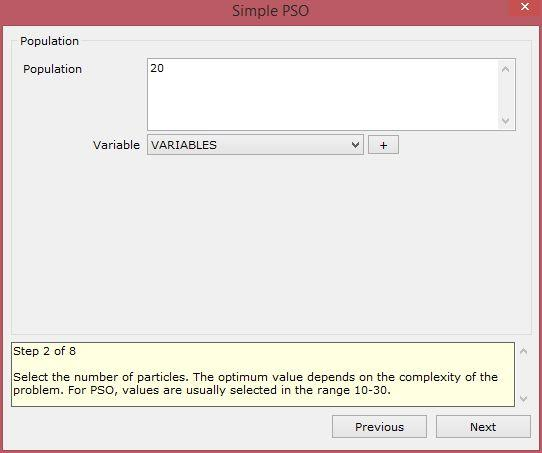Select the number of particles. The optimum value depends on the complexity of the problem. For PSO, values are usually selected in the range 10-30.

The variables that may be used in the expression are:

• VARIABLES: the number of variables.

#### Step 3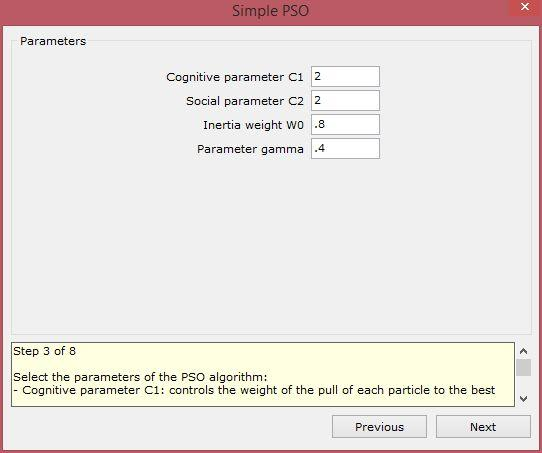Select the parameters of the PSO algorithm:

• Cognitive parameter C1: controls the weight of the pull of each particle to the best ever position of the same particle. Usual value for simple PSO is 2.
• Social parameter C2: controls the weight of the pull of each particle to the best ever position of the whole swarm. Usual value for simple PSO is 2.
• Inertia parameter W0: controls the inertia of the particle. Usual values for simple PSO are in the range 0.8-1.4.
• Parameter gamma: controls the initial maximum velocities of the particles, as the fraction of the design space (per variable) that can be traveled in one time step. Usual value is 0.4.

#### Step 4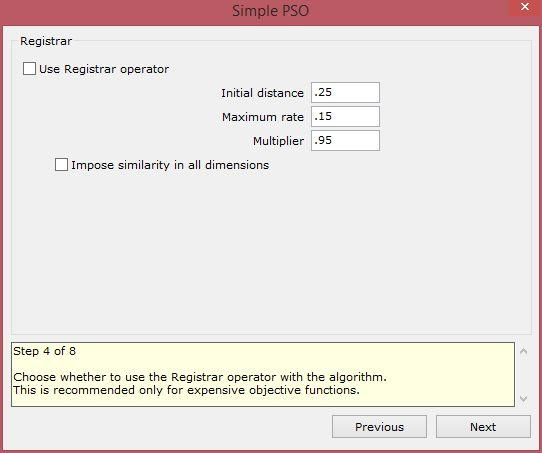Choose whether to use the Registrar operator with the algorithm. This is recommended only for expensive objective functions.

#### Step 5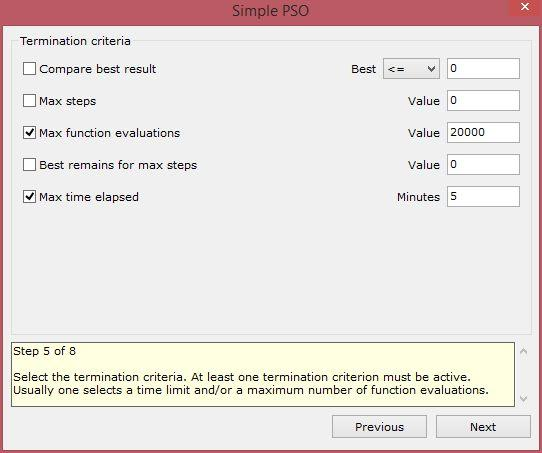Select the termination criteria. At least one termination criterion must be active. Usually one selects a maximum number of function evaluations and/or a time limit.

#### Step 6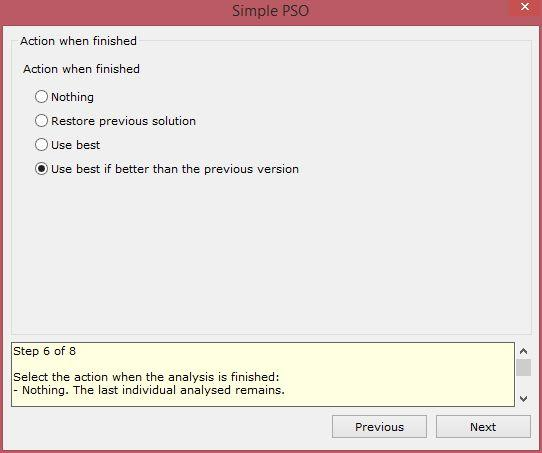Select the action when the analysis is finished:

• Nothing: The last individual analysed remains.
• Restore previous solution: The solution that was visible before the analysis is restored.
• Use best: The best solution that was discovered during the analysis is applied.
• Use best if better than the previous version (recommended): The best solution that was discovered during the analysis is applied only if it is better than the previous solution. In the opposite case the previous solution is restored.

#### Step 7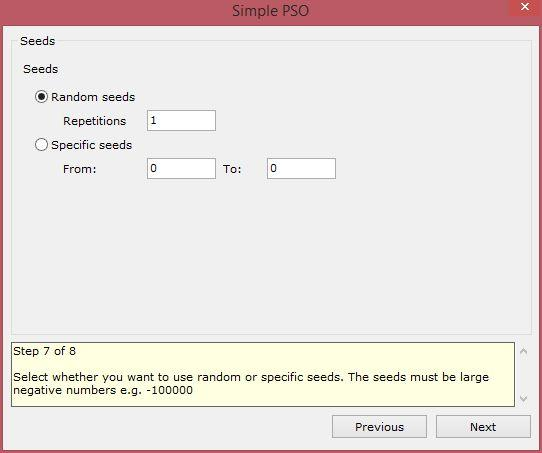Select whether you want to use random or specific seeds for the random number generator. The seeds must be large negative numbers e.g. -100000. Usually a number of analyses with random seeds is performed. The filename of the log is the seed used in the specific analysis.

#### Step 8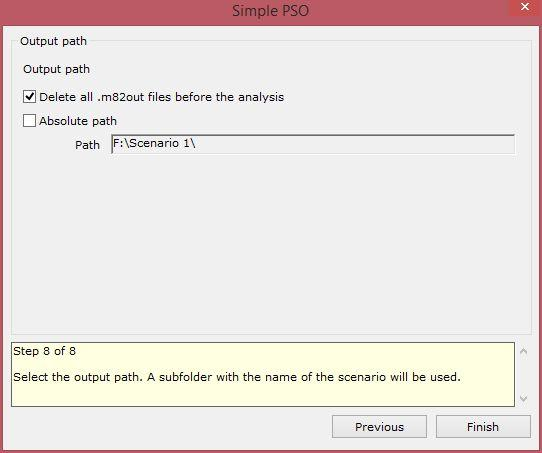Select the output path. A subfolder with the name of the scenario will be used. Usually a relative path is used i.e. the absolute path check box is not checked. This results in the output logs being saved in the same path as the project file.